# Thermodynamics, part 3.ppt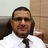- Assist.Prof of Laser Physics & Photonics en Ibb University, Yemen + Jazan University, KSA
2 de Sep de 2016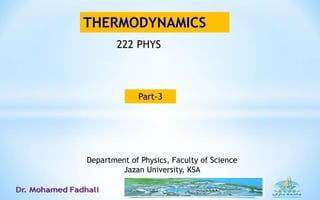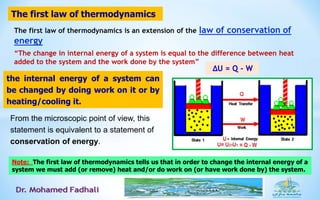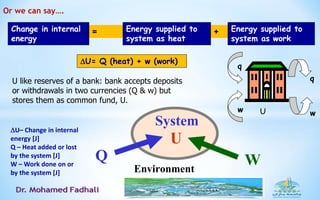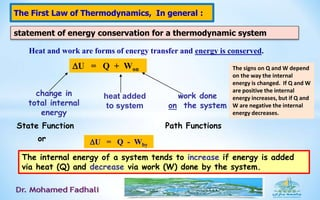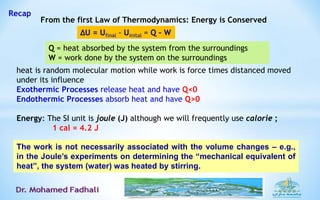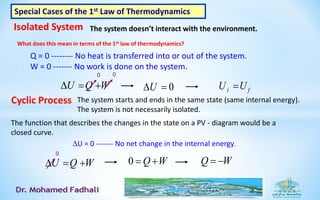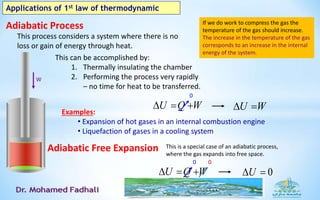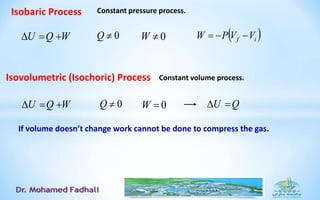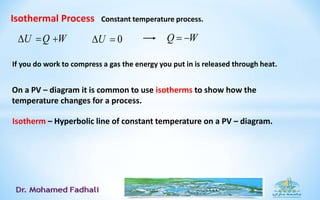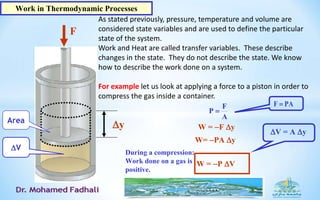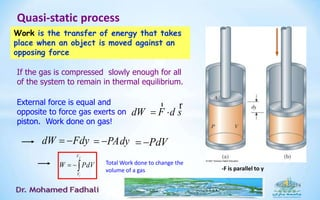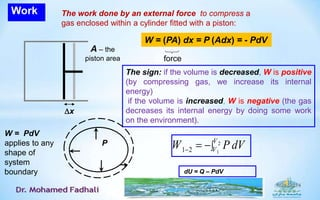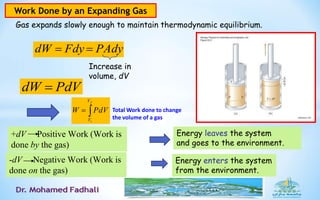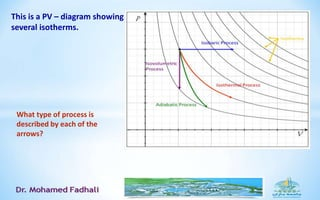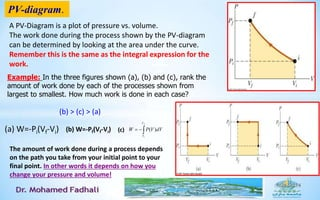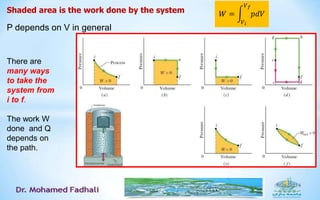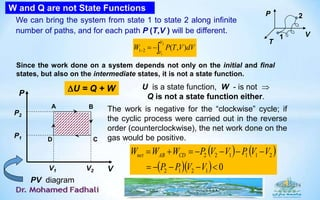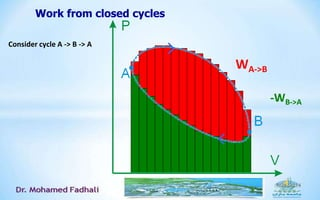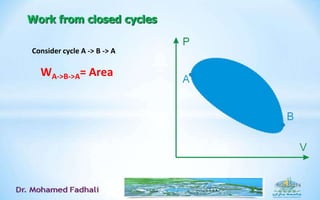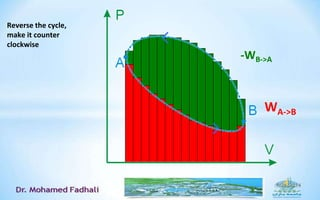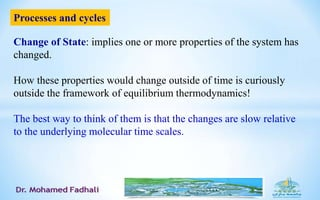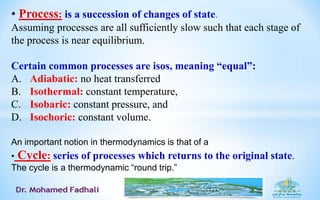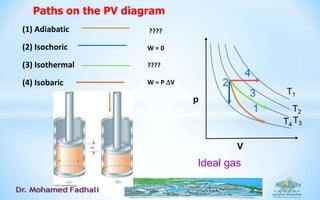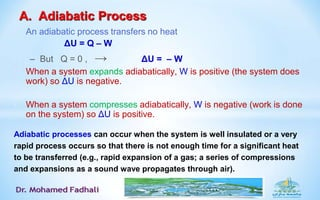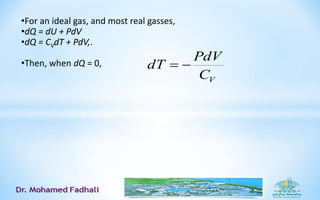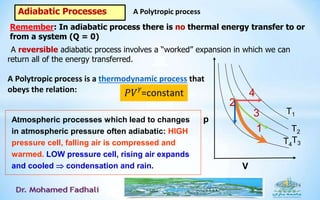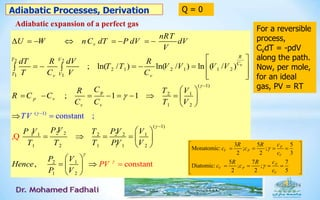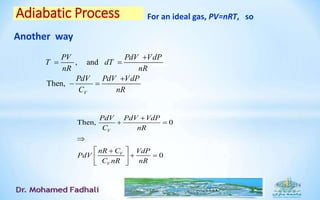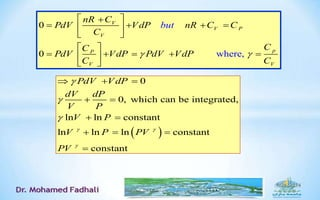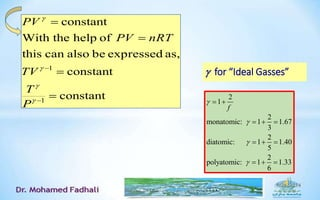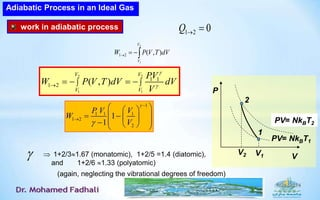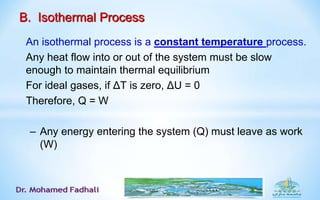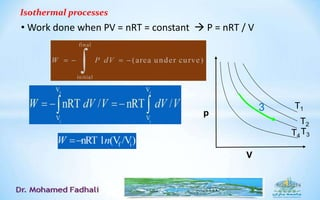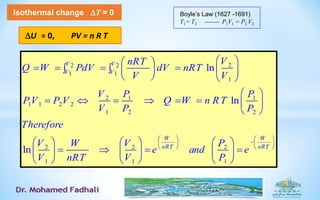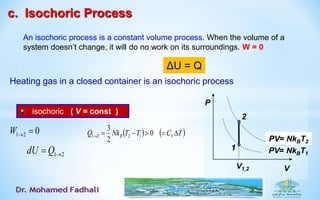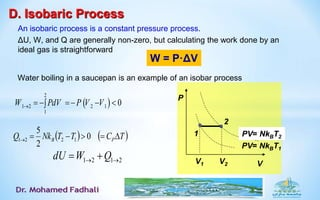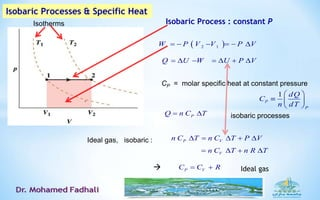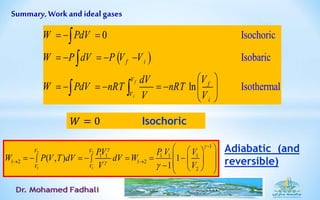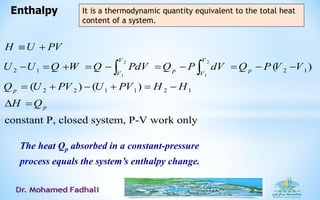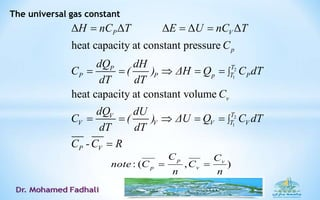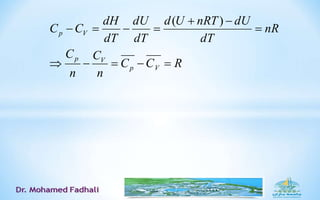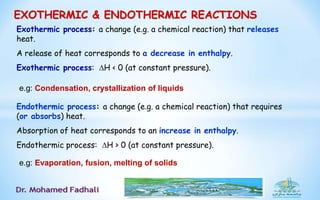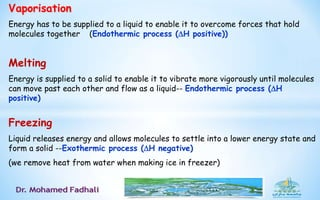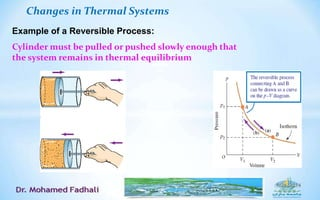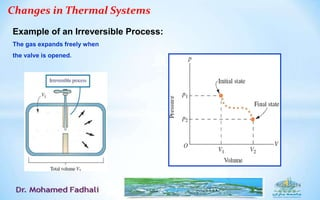1 de 45

### Thermodynamics, part 3.ppt

• 1. THERMODYNAMICS Department of Physics, Faculty of Science Jazan University, KSA Part-3 222 PHYS
• 2. The first law of thermodynamics is an extension of the law of conservation of energy “The change in internal energy of a system is equal to the difference between heat added to the system and the work done by the system” The first law of thermodynamics the internal energy of a system can be changed by doing work on it or by heating/cooling it. From the microscopic point of view, this statement is equivalent to a statement of conservation of energy. Note: The first law of thermodynamics tells us that in order to change the internal energy of a system we must add (or remove) heat and/or do work on (or have work done by) the system. ΔU = Q - W
• 3. +=Change in internal energy Energy supplied to system as heat Energy supplied to system as work U= Q (heat) + w (work) q w q wU Or we can say…. U like reserves of a bank: bank accepts deposits or withdrawals in two currencies (Q & w) but stores them as common fund, U. System U Environment Q W U– Change in internal energy [J] Q – Heat added or lost by the system [J] W – Work done on or by the system [J]
• 4. Heat and work are forms of energy transfer and energy is conserved. The First Law of Thermodynamics, In general : U = Q + Won work done on the system change in total internal energy heat added to system U = Q - Wby State Function Path Functions statement of energy conservation for a thermodynamic system The internal energy of a system tends to increase if energy is added via heat (Q) and decrease via work (W) done by the system. or The signs on Q and W depend on the way the internal energy is changed. If Q and W are positive the internal energy increases, but if Q and W are negative the internal energy decreases.
• 5. heat is random molecular motion while work is force times distanced moved under its influence Exothermic Processes release heat and have Q<0 Endothermic Processes absorb heat and have Q>0 Energy: The SI unit is joule (J) although we will frequently use calorie ; 1 cal = 4.2 J Recap The work is not necessarily associated with the volume changes – e.g., in the Joule’s experiments on determining the “mechanical equivalent of heat”, the system (water) was heated by stirring. ΔU = Ufinal - Uinital = Q – W From the first Law of Thermodynamics: Energy is Conserved Q = heat absorbed by the system from the surroundings W = work done by the system on the surroundings
• 6. Special Cases of the 1st Law of Thermodynamics Isolated System The system doesn’t interact with the environment. What does this mean in terms of the 1st law of thermodynamics? Q = 0 -------- No heat is transferred into or out of the system. W = 0 ------- No work is done on the system. U Q W   0 0 0U  i fU U Cyclic Process The system starts and ends in the same state (same internal energy). The system is not necessarily isolated. The function that describes the changes in the state on a PV - diagram would be a closed curve. U = 0 ------- No net change in the internal energy. U Q W   0 WQ 0 WQ 
• 7. Adiabatic Process W This process considers a system where there is no loss or gain of energy through heat. This can be accomplished by: 1. Thermally insulating the chamber 2. Performing the process very rapidly – no time for heat to be transferred. Examples: • Expansion of hot gases in an internal combustion engine • Liquefaction of gases in a cooling system Adiabatic Free Expansion This is a special case of an adiabatic process, where the gas expands into free space. U Q W   0 U W  U Q W   0 0 0U  If we do work to compress the gas the temperature of the gas should increase. The increase in the temperature of the gas corresponds to an increase in the internal energy of the system. Applications of 1st law of thermodynamic
• 8. Isobaric Process Constant pressure process. U Q W   0Q 0W  if VVPW  Isovolumetric (Isochoric) Process Constant volume process. U Q W   0Q 0W If volume doesn’t change work cannot be done to compress the gas. U Q 
• 9. Isothermal Process U Q W   0U  WQ  Constant temperature process. If you do work to compress a gas the energy you put in is released through heat. On a PV – diagram it is common to use isotherms to show how the temperature changes for a process. Isotherm – Hyperbolic line of constant temperature on a PV – diagram.
• 10. F W = F y A F P  PAF  W= PA y V = A y W = P V y V Area Work in Thermodynamic Processes During a compression: Work done on a gas is positive. As stated previously, pressure, temperature and volume are considered state variables and are used to define the particular state of the system. Work and Heat are called transfer variables. These describe changes in the state. They do not describe the state. We know how to describe the work done on a system. For example let us look at applying a force to a piston in order to compress the gas inside a container.
• 11. External force is equal and opposite to force gas exerts on piston. Work done on gas! dW F d s  r r PAdy  PdVFdydW   f i V V PdVW -F is parallel to y Total Work done to change the volume of a gas If the gas is compressed slowly enough for all of the system to remain in thermal equilibrium. Quasi-static process Work is the transfer of energy that takes place when an object is moved against an opposing force
• 12. Work The sign: if the volume is decreased, W is positive (by compressing gas, we increase its internal energy) if the volume is increased, W is negative (the gas decreases its internal energy by doing some work on the environment). 2 11 2 V VW P dV   The work done by an external force to compress a gas enclosed within a cylinder fitted with a piston: W = (PA) dx = P (Adx) = - PdV x P W = PdV applies to any shape of system boundary dU = Q – PdV A – the piston area force
• 13. Work Done by an Expanding Gas Gas expands slowly enough to maintain thermodynamic equilibrium. +dV Positive Work (Work is done by the gas) -dV Negative Work (Work is done on the gas) Energy leaves the system and goes to the environment. Energy enters the system from the environment. PAdyFdydW  Increase in volume, dV PdVdW   f i V V PdVW Total Work done to change the volume of a gas
• 14. What type of process is described by each of the arrows? This is a PV – diagram showing several isotherms.
• 15. A PV-Diagram is a plot of pressure vs. volume. The work done during the process shown by the PV-diagram can be determined by looking at the area under the curve. Remember this is the same as the integral expression for the work. Example: In the three figures shown (a), (b) and (c), rank the amount of work done by each of the processes shown from largest to smallest. How much work is done in each case? (b) > (c) > (a) The amount of work done during a process depends on the path you take from your initial point to your final point. In other words it depends on how you change your pressure and volume! (a) W=-Pi(Vf-Vi) (b) W=-Pf(Vf-Vi) (c)  f i V V dVVPW )( PV-diagram.
• 16. Shaded area is the work done by the system P depends on V in general There are many ways to take the system from i to f. The work W done and Q depends on the path. 𝑊 = 𝑉 𝑖 𝑉 𝑓 𝑝𝑑𝑉
• 17. W and Q are not State Functions P V P2 P1 V1 V2 A B CD The work is negative for the “clockwise” cycle; if the cyclic process were carried out in the reverse order (counterclockwise), the net work done on the gas would be positive.        01212 211122   VVPP VVPVVPWWW CDABnet  2 1 ),(21 V V dVVTPW We can bring the system from state 1 to state 2 along infinite number of paths, and for each path P (T,V ) will be different. U is a state function, W - is not  Q is not a state function either. U = Q + W Since the work done on a system depends not only on the initial and final states, but also on the intermediate states, it is not a state function. PV diagram P V T 1 2
• 18. Work from closed cycles Consider cycle A -> B -> A WA->B -WB->A
• 19. Work from closed cycles Consider cycle A -> B -> A WA->B->A= Area
• 20. Reverse the cycle, make it counter clockwise -WB->A WA->B
• 21. Change of State: implies one or more properties of the system has changed. How these properties would change outside of time is curiously outside the framework of equilibrium thermodynamics! The best way to think of them is that the changes are slow relative to the underlying molecular time scales. Processes and cycles
• 22. • Process: is a succession of changes of state. Assuming processes are all sufficiently slow such that each stage of the process is near equilibrium. Certain common processes are isos, meaning “equal”: A. Adiabatic: no heat transferred B. Isothermal: constant temperature, C. Isobaric: constant pressure, and D. Isochoric: constant volume. An important notion in thermodynamics is that of a • Cycle: series of processes which returns to the original state. The cycle is a thermodynamic “round trip.”
• 23. (1) Adiabatic (2) Isochoric (3) Isothermal (4) Isobaric Paths on the PV diagram p V 2 1 3 4 T1 T2 T3T4 ???? W = 0 ???? W = P V Ideal gas
• 24. A. Adiabatic Process • An adiabatic process transfers no heat ΔU = Q – W – But Q = 0 , → ΔU = – W • When a system expands adiabatically, W is positive (the system does work) so ΔU is negative. • When a system compresses adiabatically, W is negative (work is done on the system) so ΔU is positive. Adiabatic processes can occur when the system is well insulated or a very rapid process occurs so that there is not enough time for a significant heat to be transferred (e.g., rapid expansion of a gas; a series of compressions and expansions as a sound wave propagates through air).
• 25. •For an ideal gas, and most real gasses, •dQ = dU + PdV •dQ = CVdT + PdV,. •Then, when dQ = 0, VC PdV dT 
• 26. Adiabatic Processes Remember: In adiabatic process there is no thermal energy transfer to or from a system (Q = 0) A reversible adiabatic process involves a “worked” expansion in which we can return all of the energy transferred. A Polytropic process is a thermodynamic process that obeys the relation: p V 2 1 3 4 T1 T2 T3T4 Atmospheric processes which lead to changes in atmospheric pressure often adiabatic: HIGH pressure cell, falling air is compressed and warmed. LOW pressure cell, rising air expands and cooled  condensation and rain. A Polytropic process
• 27. 2 2 2 1 2 1 1 2 1 1 ( 1) p 2 1 1 2 ( 1) 2 21 1 2 2 2 1 1 2 1 1 1 ( 1) 2 ; ln( / ) ln( / ) ln ( / ) ; 1 constant , ; 1 v R T V Cv T Vv v p v v v nRT U W nC dT P dV dV V dT R dV R T T V V V V T C V C C T VR R C C C C T V TV P VP V T PV V T T T PV V Henc                                                       Q 2 1 1 2 cons an, t t P V e P V V P          Q = 0Adiabatic Processes, Derivation 3 5 5 Monatomic: ; ; 2 2 3 5 7 7 Diatomic: ; ; 2 2 5 P V P V P V P V cR R c c c cR R c c c                     Adiabatic expansion of a perfect gas For a reversible process, CVdT = -pdV along the path. Now, per mole, for an ideal gas, PV = RT
• 28. Thermo & Stat Mech - Spring 2006 Class 3 Adiabatic Process , and Then, V PV PdV VdP T dT nR nR PdV PdV VdP C nR       For an ideal gas, PV=nRT, so Then, 0 0 V V V PdV PdV VdP C nR nR C VdP PdV C nR nR            Another way
• 29. Thermo & Stat Mech - Spring 2006 Class 3 29 whe 0 0 re, V V P V pP V V nR C PdV VdP nR C C C CC PdV VdP PdV VdP C C but                        0 0, which can be integrated, ln ln constant ln ln ln constant constant PdV VdP dV dP V P V P V P PV PV                 
• 30. Thermo & Stat Mech - Spring 2006 Class 3 30 constant constant as,expressedbealsocanthis ofhelpWith the constant 1 1           P T TV nRTPV PV  for “Ideal Gasses” 2 1 2 monatomic: 1 1.67 3 2 diatomic: 1 1.40 5 2 polyatomic: 1 1.33 6 f               
• 31. Adiabatic Process in an Ideal Gas work in adiabatic process 021 Q  2 1 ),(21 V V dVTVPW V P V1 PV= NkBT1 PV= NkBT2 1 2 V2 2 2 1 1 1 1 1 2 ( , ) V V V V PV W P V T dV dV V         1+2/31.67 (monatomic), 1+2/5 =1.4 (diatomic), and 1+2/6 1.33 (polyatomic)  (again, neglecting the vibrational degrees of freedom) 1 1 1 1 1 2 2 1 1 P V V W V                
• 32. B. Isothermal Process • An isothermal process is a constant temperature process. • Any heat flow into or out of the system must be slow enough to maintain thermal equilibrium • For ideal gases, if ΔT is zero, ΔU = 0 • Therefore, Q = W – Any energy entering the system (Q) must leave as work (W)
• 33. Isothermal processes • Work done when PV = nRT = constant  P = nRT / V p V 3 T1 T2 T3T4
• 34. Isothermal change T = 0 U = 0, PV = n R T 22 2 1 1 1 2 1 1 1 1 2 2 1 2 2 2 2 2 1 1 1 ln ln ln V V V V W W nRT nRT VnRT Q W PdV dV nRT V V V P P PV P V Q W n RT V P P Therefore V V PW e and e V nRT V P                                                             Boyle’s Law (1627 -1691) T1= T2 ------- P1V1 = P2 V2
• 35. c. Isochoric Process • An isochoric process is a constant volume process. When the volume of a system doesn’t change, it will do no work on its surroundings. W = 0 ΔU = Q Heating gas in a closed container is an isochoric process isochoric ( V = const ) 021 W    TCTTNkQ VB  0 2 3 1221 21 QdU V P V1,2 PV= NkBT1 PV= NkBT2 1 2
• 36. D. Isobaric Process • An isobaric process is a constant pressure process. • ΔU, W, and Q are generally non-zero, but calculating the work done by an ideal gas is straightforward W = P·ΔV Water boiling in a saucepan is an example of an isobar process   2 1 2 2 1 1 0W PdV P V V         TCTTNkQ PB  0 2 5 1221 2121   QWdU V P V1 PV= NkBT1 PV= NkBT21 2 V2
• 37. Isobaric Process : constant P  2 1W P V V   P V   Q U W   U P V    isobaric processesPQ n C T  CP = molar specific heat at constant pressure P Vn C T n C T P V    Ideal gas, isobaric : Vn C T n R T     P VC C R  Ideal gas Isotherms 1 P P dQ C n dT        Isobaric Processes & Specific Heat
• 38. Summary, Workand ideal gases   Isochoric Isobaric Is 0 ln othermal f i f i V f V i W PdV W P dV P V V VdV W PdV nRT nRT V V                         Adiabatic (and reversible) 𝑊 = 0 Isochoric 2 2 1 1 1 1 1 1 1 1 1 1 2 2 2 1( , 1 ) V V V V PV W P V T dV dV V P V V W V                       
• 39. The heat Qp absorbed in a constant-pressure process equals the system’s enthalpy change. Enthalpy 2 2 1 1 2 1 2 1 2 2 1 1 2 1 ( ) ( ) ( ) constant P, closed system, P-V work only V V p pV V p p H U PV U U Q W Q PdV Q P dV Q P V V Q U PV U PV H H H Q                       It is a thermodynamic quantity equivalent to the total heat content of a system.
• 40. 2 1 2 1 Δ Δ Δ Δ Δ heat capacity at constant pressure heat capacity at constant volume P V p TP TP P p P v TV TV V V V P V H nC T E U nC T C dQ dH C ( ) ΔH Q C dT dT dT C dQ dU C ( ) ΔU Q C dT dT dT C -C R                 ),(: n C C n C Cnote v v p p  The universal gas constant
• 42. EXOTHERMIC & ENDOTHERMIC REACTIONS Exothermic process: a change (e.g. a chemical reaction) that releases heat. A release of heat corresponds to a decrease in enthalpy. Exothermic process: H < 0 (at constant pressure). Endothermic process: a change (e.g. a chemical reaction) that requires (or absorbs) heat. Absorption of heat corresponds to an increase in enthalpy. Endothermic process: H > 0 (at constant pressure). e.g: Evaporation, fusion, melting of solids e.g: Condensation, crystallization of liquids
• 43. Vaporisation Energy has to be supplied to a liquid to enable it to overcome forces that hold molecules together (Endothermic process (H positive)) Melting Energy is supplied to a solid to enable it to vibrate more vigorously until molecules can move past each other and flow as a liquid-- Endothermic process (H positive) Freezing Liquid releases energy and allows molecules to settle into a lower energy state and form a solid --Exothermic process (H negative) (we remove heat from water when making ice in freezer)
• 44. Changes in Thermal Systems Example of a Reversible Process: Cylinder must be pulled or pushed slowly enough that the system remains in thermal equilibrium
• 45. Changes in Thermal Systems Example of an Irreversible Process: The gas expands freely when the valve is opened.

1. 28
2. 29
3. 30